# ISRO Scientist or Engineer Electrical 2018

Instructions

For the following questions answer them individually

Question 1

# For the system to be stable, in a negative feedback systems, with increase of feedback loops the range of gain (K)Question 2

# What is the impulse response for the system indicated below?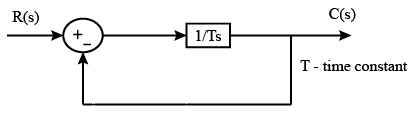Question 3

# The open loop transfer function of a unity feedback control system is $$G(s) = \frac{K}{(s + 2)(s + 1)(s^2 + 6s + 25)}$$. Determine the range of gain ‘K’ for the unity feedback control system to be stable.Question 4

# For the step response $$C(s) = \frac{10(s - 2)}{s(s^2 + 4s + 5)}$$ find initial and final value of C(s).Question 5

# What is the condition for the below system to be critically damped?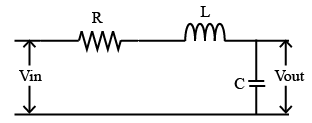Question 6

# Transfer function of two compensators are:$$C_1 = \frac{100(S + 3)}{(S + 200)}$$ $$C_2 = \frac{S + 200}{100(S + 3)}$$Which of the following statement is correct?Question 7

# In the system shown below, what is steady state error in unit ramp response?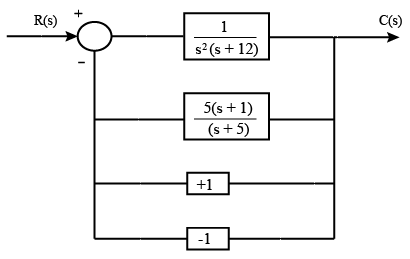Question 8

# Typical root locus diagram of a system is shown below. Find the point where the system is critically damped.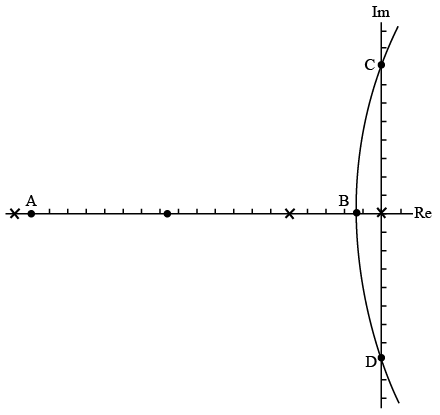Question 9

# If a network consists of ‘n’ number of principle nodes and ‘b’ numbers of branches. Then, mesh analysis becomes simpler than nodal analysis if ‘n’ is greater thanQuestion 10

# What is the equivalent resistance between the terminals A and B ?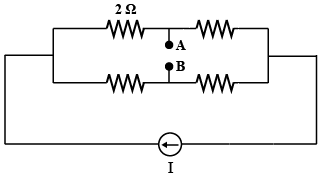OR